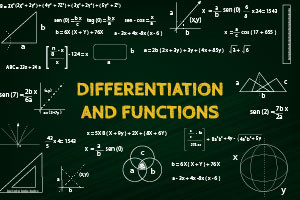# Differentiation and Functions in Mathematics - Revised

## Gain a good understanding of differentiation and functions in mathematics.

Calculus
Free Course
In mathematics functions are the idealization of how a varying quantity depends on another quantity, and Differentiation allows you to find and show rates of change, the two work hand-in-hand. Study this course to understand how to calculate the differentiation, of a function, how to plot your functions on graphs, applications of differentiation, integration and more.

2-3 Hours

Yes

Yes

Yes
• ### Publisher## Description

In mathematics functions are the idealization of how a varying quantity depends on another quantity, and Differentiation allows you to find and show rates of change, the two work hand-in-hand. This course will give you a detailed insight to both functions and differentiation, and how to apply them for solving mathematical problems, and questions.

The course starts off with teaching you how to plot equations from a table of values on a graph, form quadratics to exponentials. You will learn about the algebra technique “completing the square” the process of translating functions on the X and Y axis and how to stretch them on both axis. Along with what the discriminant is and how to calculate it, and what inverse and Bijective functions are.
In the second module you will be introduced to basic differentiation and how to differentiate polynomials. You will learn about the equation of a tangent and a normal of a curve, what the second derivative is and how to derive it, differentiation of tangents, products and quotients. The module finishes by teaching you the Chain Rule, the Product Rule, and the Quotient Rule.

Next the course teaches you about the differentiation of logarithms, and how to differentiate trigonometry equations. After which the module discusses the relationship between differentiation and the rates of change, giving you two working examples. You will learn about using differentiation to calculate the turning points on a curve, the applications of differentiation in regards to velocity, acceleration, and electricity. Then the module moves on to showing you how to work out an algebraic formula from just two measurements, and exploring the relationship between different types of functions, their differential, and second derivative.

In the last topic you are introduced to Integration, in mathematics integration is the reverse of differentiation. You will learn about what it is at a basic level, integrating different types of formulas, and how to deal with the powers, indices and fractions for integration. You will be though about what definite integration is and integration with trigonometry and exponential equations.

Start Course Now

## Learning Outcomes

Having completed this course students will be able to:

• Identify how to plot Quadratic, cubic, reciprocal and exponential graphs from a table of values
• Identify how to translate or stretch a function along either the x or y axis
• Identify an inverse function and a bijective function
• Recognize how to calculate the gradient of a curve
• Calculate the differentiation of several different types of formulas and equations
• Identify the chain rule, the product rule and the quotient rule
• Calculate the turning points of a curve
• Use differentiation to calculate the displacement, velocity and acceleration, of an object
• Discuss the relationships between different equations/functions and their differentials
• Discuss what basic integration is and calculate it
• Define and calculate definite integration
• Calculate the area under a curve with integration

## Certification

All Alison courses are free to enrol, study and complete. To successfully complete this Certificate course and become an Alison Graduate, you need to achieve 80% or higher in each course assessment. Once you have completed this Certificate course, you have the option to acquire official Certification, which is a great way to share your achievement with the world. Your Alison Certification is:

Ideal for sharing with potential employers - include it in your CV, professional social media profiles and job applications
An indication of your commitment to continuously learn, upskill and achieve high results
An incentive for you to continue empowering yourself through lifelong learning

Alison offers 3 types of Certification for completed Certificate courses:

Digital Certificate - a downloadable Certificate in PDF format, immediately available to you when you complete your purchase
Certificate - a physical version of your officially branded and security-marked Certificate, posted to you with FREE shipping
Framed Certificate - a physical version of your officially branded and security-marked Certificate in a stylish frame, posted to you with FREE shipping